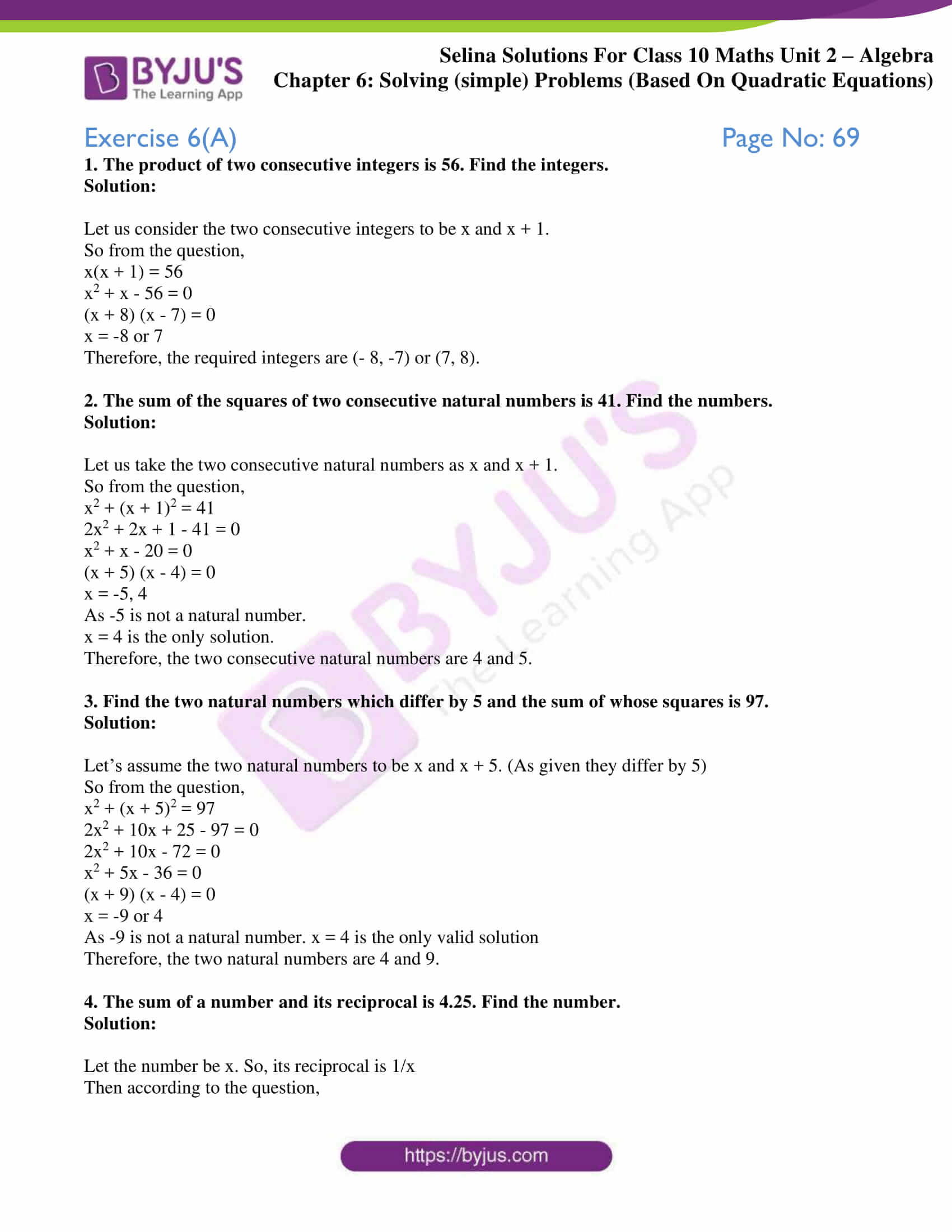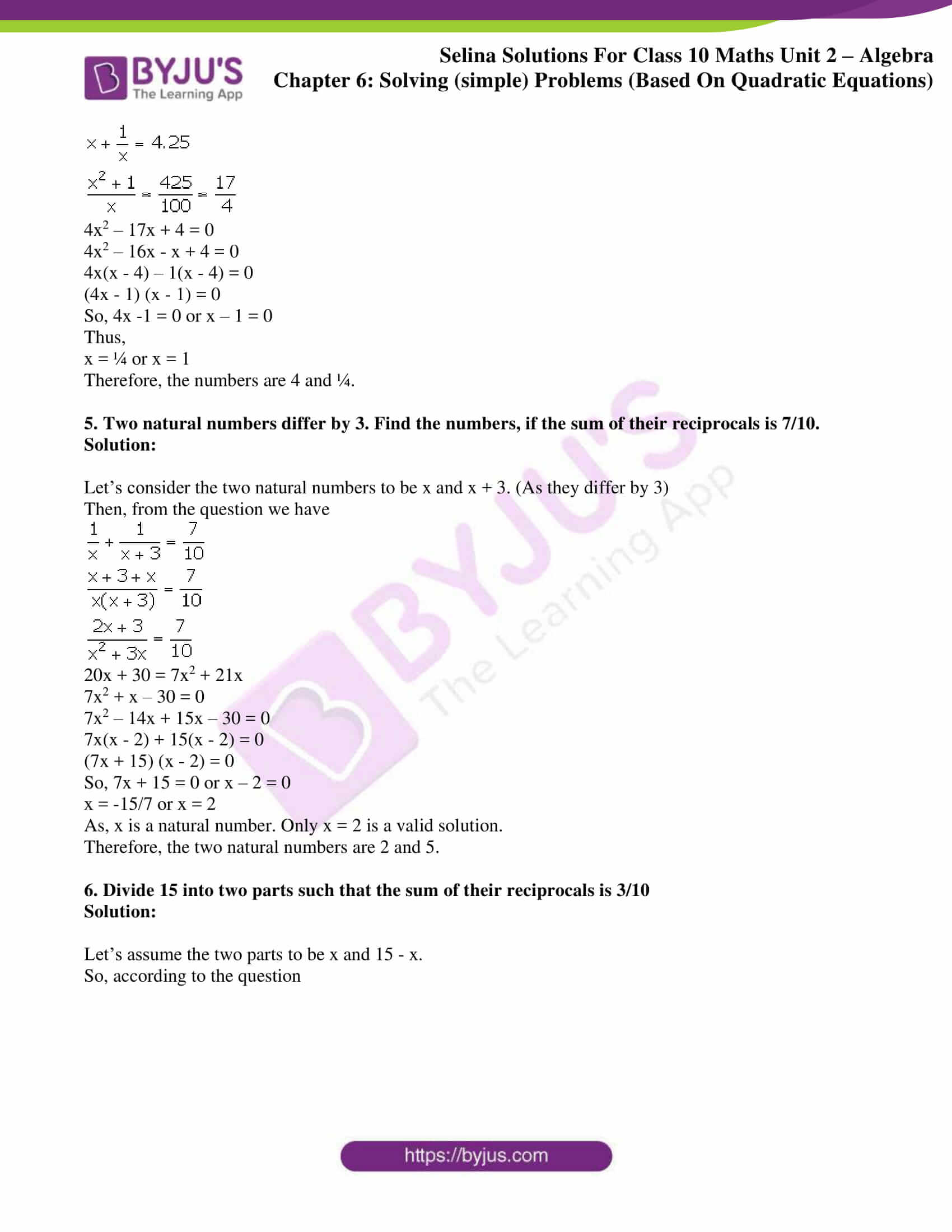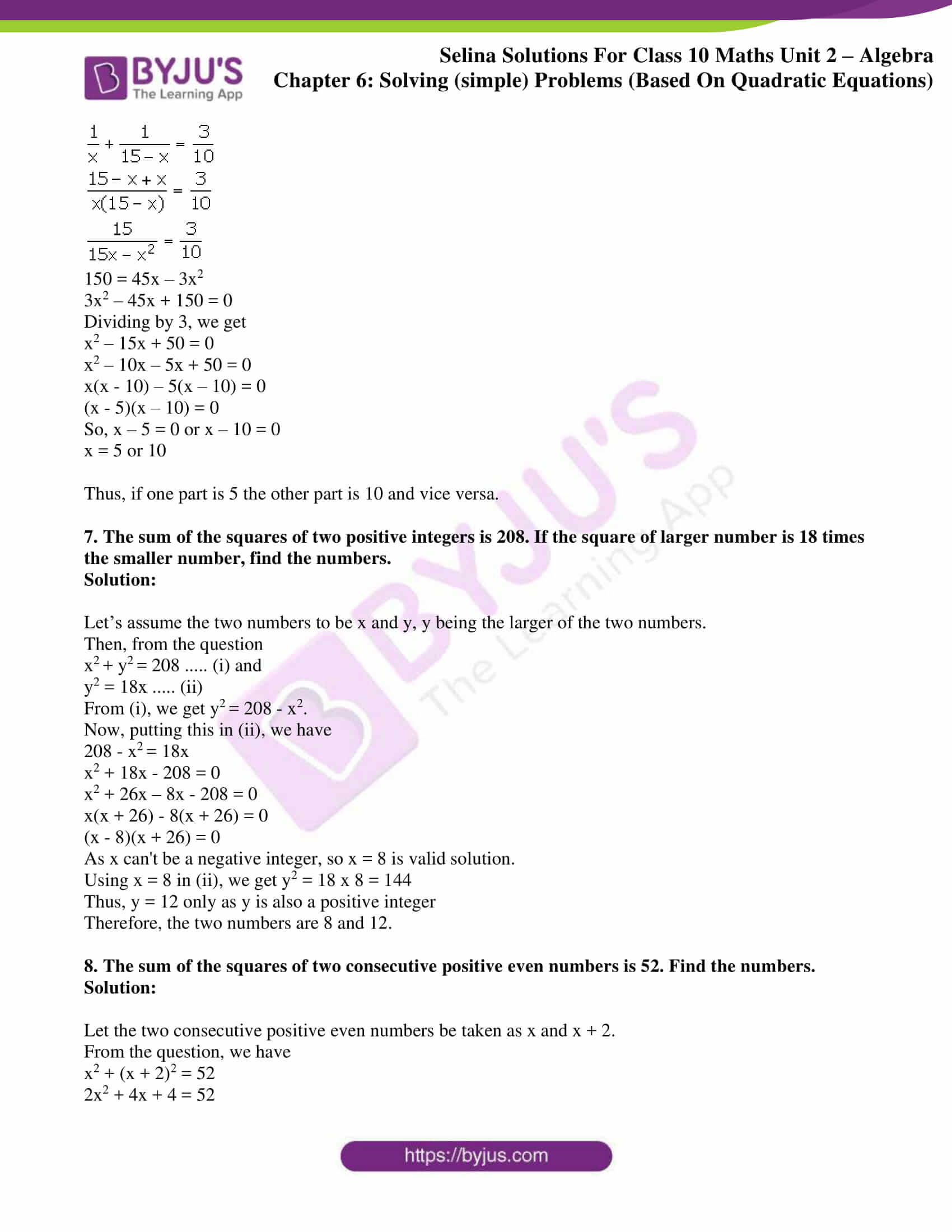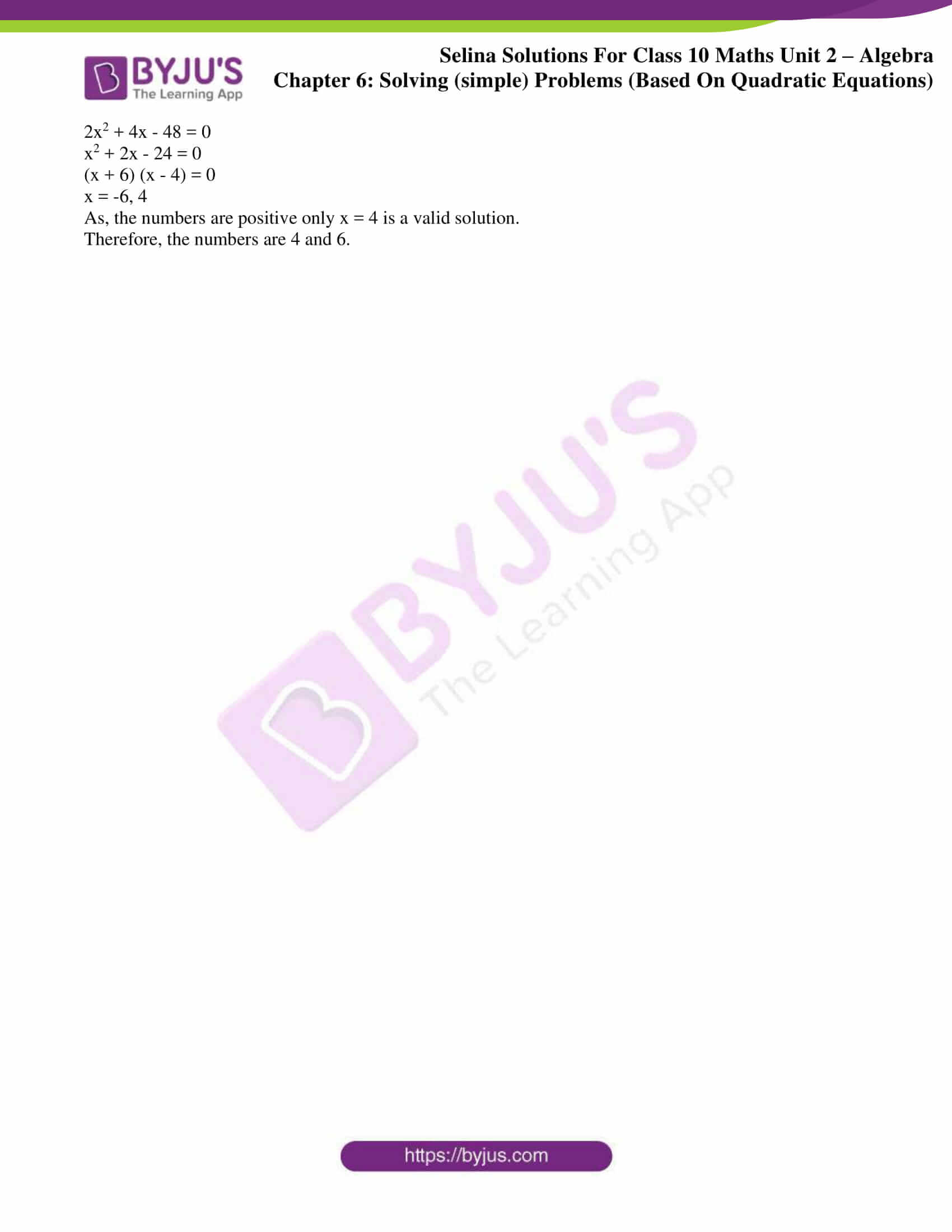# Selina Solutions Concise Maths Class 10 Chapter 6 Solving (simple) Problems (Based On Quadratic Equations) Exercise 6(A)

After understanding how to solve quadratic equations, the next step is to solve other word problems. Problems based on numbers and based on time and work are the two main concepts covered in this exercise. The Selina Solutions for Class 10 Maths can be used by students to clear any doubts and also learn the right way to solve problems. Students can also access the Concise Selina Solutions for Class 10 Maths Chapter 6 Solving (simple) Problems (Based On Quadratic Equations) Exercise 6(A) PDF, in the links below.

## Selina Solutions Concise Maths Class 10 Chapter 6 Solving (simple) Problems (Based On Quadratic Equations) Exercise 6(A) Download PDF### Access Selina Solutions Concise Maths Class 10 Chapter 6 Solving (simple) Problems (Based On Quadratic Equations) Exercise 6(A)

1. The product of two consecutive integers is 56. Find the integers.

Solution:

Let us consider the two consecutive integers to be x and x + 1.

So from the question,

x(x + 1) = 56

x2 + x – 56 = 0

(x + 8) (x – 7) = 0

x = -8 or 7

Therefore, the required integers are (- 8, -7) or (7, 8).

2. The sum of the squares of two consecutive natural numbers is 41. Find the numbers.

Solution:

Let us take the two consecutive natural numbers as x and x + 1.

So from the question,

x2 + (x + 1)2 = 41

2x2 + 2x + 1 – 41 = 0

x2 + x – 20 = 0

(x + 5) (x – 4) = 0

x = -5, 4

As -5 is not a natural number.

x = 4 is the only solution.

Therefore, the two consecutive natural numbers are 4 and 5.

3. Find the two natural numbers which differ by 5 and the sum of whose squares is 97.

Solution:

Let’s assume the two natural numbers to be x and x + 5. (As given they differ by 5)

So from the question,

x2 + (x + 5)2 = 97

2x2 + 10x + 25 – 97 = 0

2x2 + 10x – 72 = 0

x2 + 5x – 36 = 0

(x + 9) (x – 4) = 0

x = -9 or 4

As -9 is not a natural number. x = 4 is the only valid solution

Therefore, the two natural numbers are 4 and 9.

4. The sum of a number and its reciprocal is 4.25. Find the number.

Solution:

Let the number be x. So, its reciprocal is 1/x

Then according to the question,4x2 – 17x + 4 = 0

4x2 – 16x – x + 4 = 0

4x(x – 4) – 1(x – 4) = 0

(4x – 1) (x – 1) = 0

So, 4x -1 = 0 or x – 1 = 0

Thus,

x = ¼ or x = 1

Therefore, the numbers are 4 and ¼.

5. Two natural numbers differ by 3. Find the numbers, if the sum of their reciprocals is 7/10.

Solution:

Let’s consider the two natural numbers to be x and x + 3. (As they differ by 3)

Then, from the question we have20x + 30 = 7x2 + 21x

7x2 + x – 30 = 0

7x2 – 14x + 15x – 30 = 0

7x(x – 2) + 15(x – 2) = 0

(7x + 15) (x – 2) = 0

So, 7x + 15 = 0 or x – 2 = 0

x = -15/7 or x = 2

As, x is a natural number. Only x = 2 is a valid solution.

Therefore, the two natural numbers are 2 and 5.

6. Divide 15 into two parts such that the sum of their reciprocals is 3/10

Solution:

Let’s assume the two parts to be x and 15 – x.

So, according to the question150 = 45x – 3x2

3x2 – 45x + 150 = 0

Dividing by 3, we get

x2 – 15x + 50 = 0

x2 – 10x – 5x + 50 = 0

x(x – 10) – 5(x – 10) = 0

(x – 5)(x – 10) = 0

So, x – 5 = 0 or x – 10 = 0

x = 5 or 10

Thus, if one part is 5 the other part is 10 and vice versa.

7. The sum of the squares of two positive integers is 208. If the square of larger number is 18 times the smaller number, find the numbers.

Solution:

Let’s assume the two numbers to be x and y, y being the larger of the two numbers.

Then, from the question

x+ y= 208 ….. (i) and

y2 = 18x ….. (ii)

From (i), we get y2 = 208 – x2.

Now, putting this in (ii), we have

208 – x= 18x

x2 + 18x – 208 = 0

x2 + 26x – 8x – 208 = 0

x(x + 26) – 8(x + 26) = 0

(x – 8)(x + 26) = 0

As x can’t be a negative integer, so x = 8 is valid solution.

Using x = 8 in (ii), we get y2 = 18 x 8 = 144

Thus, y = 12 only as y is also a positive integer

Therefore, the two numbers are 8 and 12.

8. The sum of the squares of two consecutive positive even numbers is 52. Find the numbers.

Solution:

Let the two consecutive positive even numbers be taken as x and x + 2.

From the question, we have

x2 + (x + 2)2 = 52

2x2 + 4x + 4 = 52

2x2 + 4x – 48 = 0

x2 + 2x – 24 = 0

(x + 6) (x – 4) = 0

x = -6, 4

As, the numbers are positive only x = 4 is a valid solution.

Therefore, the numbers are 4 and 6.